# How to COUNT and SUM greater than and less than a number in Excel?

In general life we usually need to count the number among a range of values based on the criteria ‘greater than a fixed value and less than another value’. We may also need to sum the total values for the matched samples. In this case. we will use COUNTIF and SUMIF function to do the calculation. And these functions are frequently used in statistic analysis.

First we want to know the basic syntax of the two functions.

```=COUNTIF (range, criterion)
=SUMIF (range, criteria, sum range)```

Assume we are in a school and statistic the scores in an exam. Here is the list for ID and Score. We use this sample to illustrate the COUNTIF function and SUMIF.

### COUNTIF Function

#1 In above assumption, we need to count the number which score is greater than a fixed value for example 60.

In a blank cell, input the formula, in this case, the range is B2:B11, the criteria is greater than 60, so we enter `=COUNTIF (B2:B11,">60").` See screenshot below.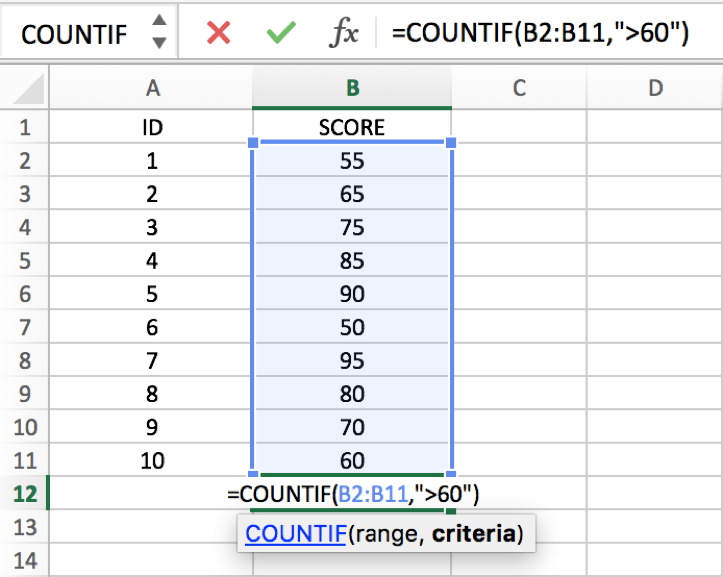After entering, the number is auto counted. And the number is 7. 60 is not included in this counting as it only equals to the criteria. If you want contains 60 in this case, just edit the criteria as “`>=60`”.

Test result ‘greater than 60’: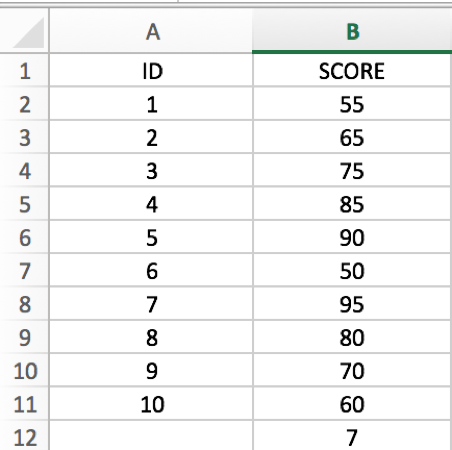Test result ‘equals and greater than 60’: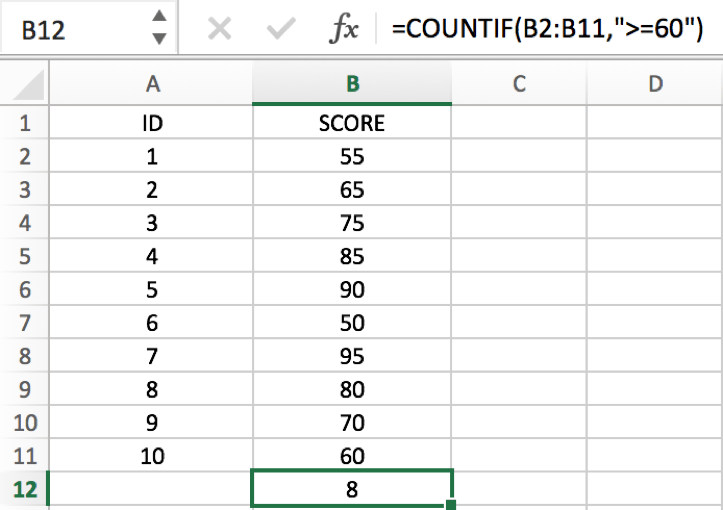#2 Count the number which score is less than a fixed value for example 80.

The same way like count ‘greater than a value’, just input `=COUNTIF(B2:B11,"<80")`.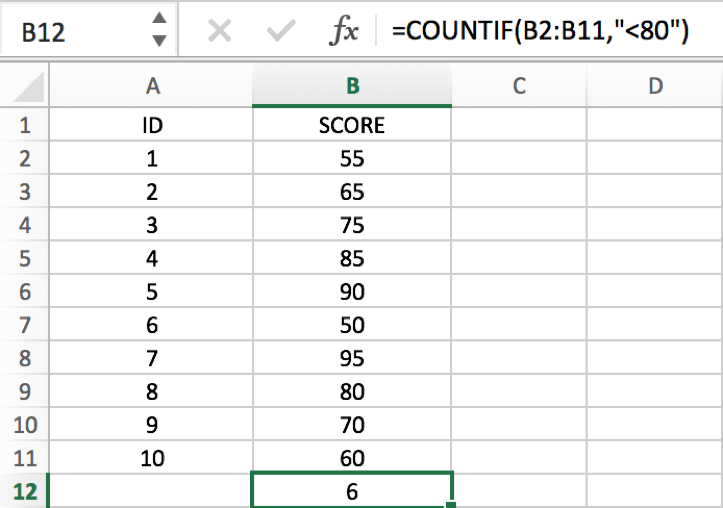#3 Now we try to count the number for score between a range like greater than 60 but less than 70.

Input formula `=COUNTIF(B2:B11,">60") - COUNTIF(B2:B11,">70")`, the range is B2:B11, but the criteria is “`>60`” and “`>70`”, be aware that for the second COUNTIF, the criteria

`>70`” means “`<=70`”, let’s see the result.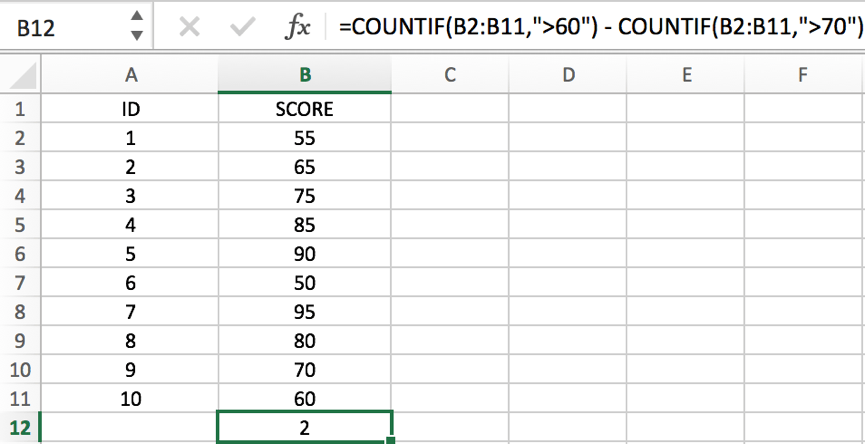65 and 70 are matched the criteria.

If we change 70 to 71, then do the same counting, only 65 matched the criteria, so then counting number is 1.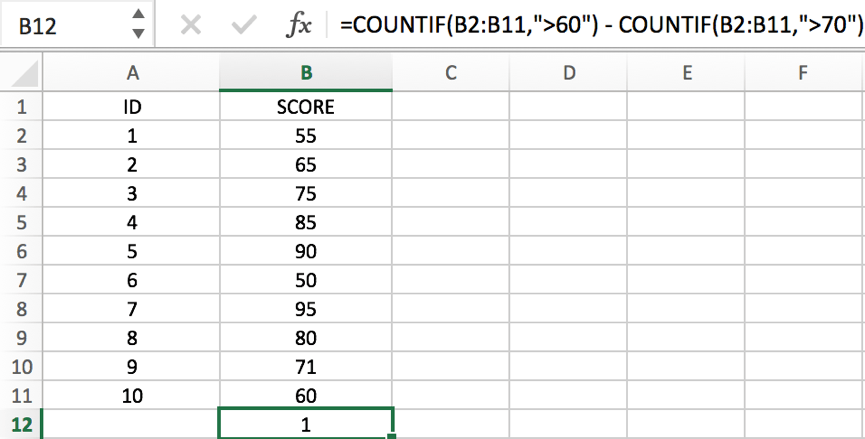### SUMIF Function

#1  In general, we also need to statistic the total score for the matched samples. So we need to use SUMIF as well. For example, we statistic the total score for those matching the criteria ‘greater than 85’.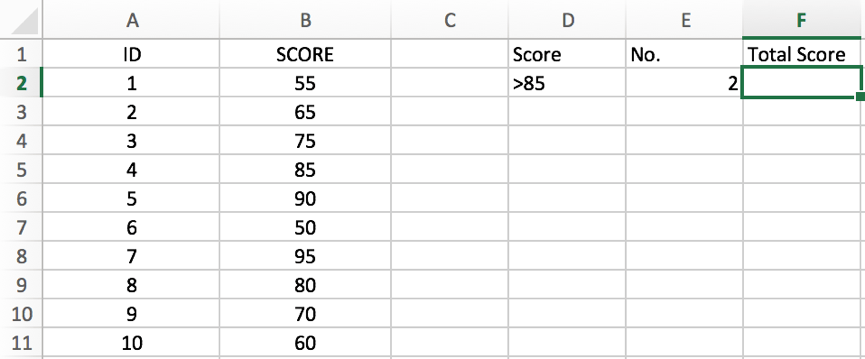Use the formula `=SUMIF(B2:B11,">85")`, then the result is loaded.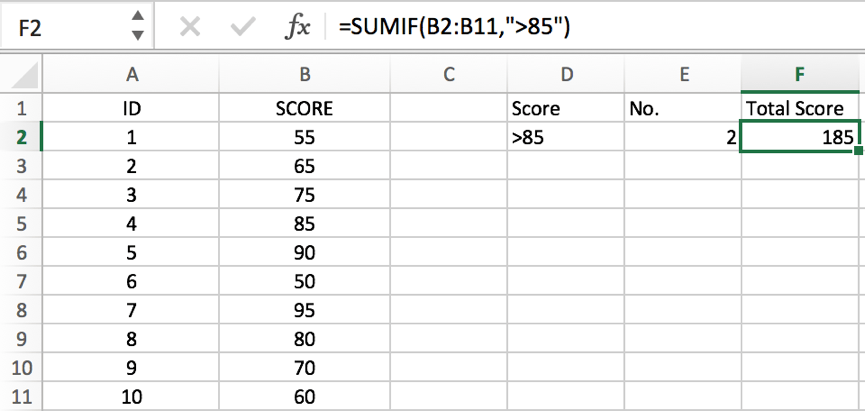#2 Sometimes we may meet cases more complex. See example below.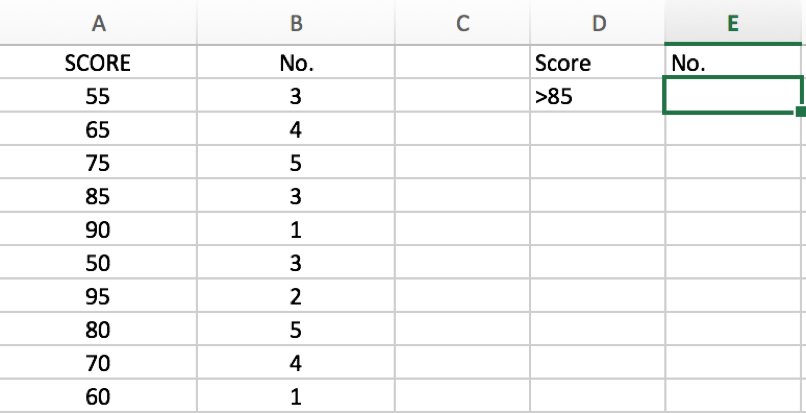In this case, there are two range. The first one is A2:A11, we use them to do criteria (filter score), the second one is B2:B11, we use them to do sum, so they are the sum range. Input the formula `=SUMIF(A2:A11,">85",B2:B11)`. See screenshot below: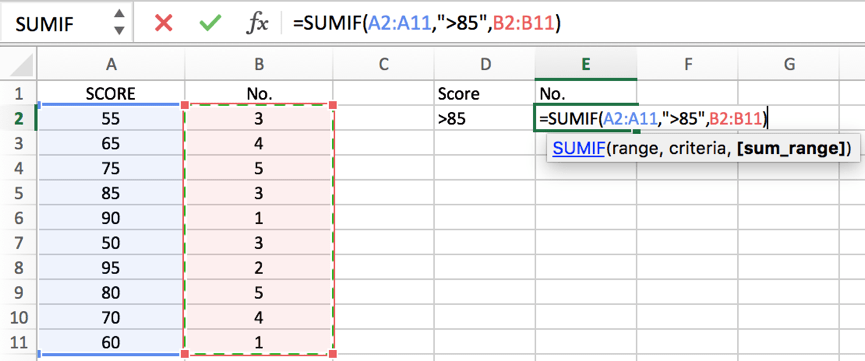Then we get the result.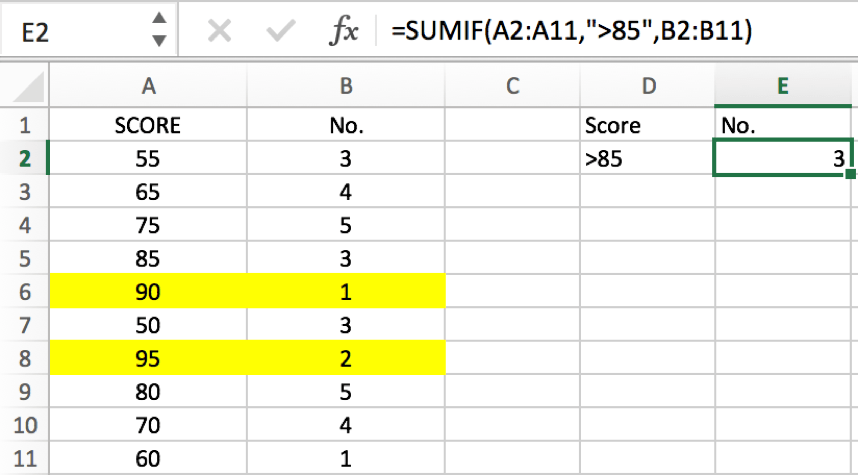### Related Functions

• Excel COUNTIF function
The Excel COUNTIF function will count the number of cells in a range that meet a given criteria. This function can be used to count the different kinds of cells with number, date, text values, blank, non-blanks, or containing specific characters.etc.= COUNTIF (range, criteria)…
• Excel SUMIF Function
The Excel SUMIF function sum the numbers in the range of cells that meet a single criteria that you specify. The syntax of the SUMIF function is as below:=SUMIF (range, criteria, [sum_range])…

Related Posts

How to Sum if Equal to Many Items or A Range in Excel

If we want to sum numbers from a range with criteria, we often select SUMIF of SUMIFS functions as the first choice to create a formula. The criteria can be a number or an array or a collection of some ...

How to Sum if Equal to X or Y in Excel

In daily work, if we want to sum numbers from a range, and only sum the numbers which being equal to X or Y in the range, we can create a formula with Excel build-in functions to get the result. ...

How to Sum if Contains an Asterisk

In our daily life, we may want to sum amounts or sales for a specific period, for example in last N days. Sum numbers in Excel is easy to run, we can apply SUM function. But if we want to ...

How to Sum if Contains an Asterisk

To add numbers together we need to apply SUM function. And if we want to add numbers based on some conditions, we can add criteria with the help of SUMIFS function, SUMIFS can filter data with multiple criteria effectively. If ...

How to Sum in Vertical Range

If we want to add numbers based on some conditions in Excel worksheet, we can add criteria with the help of SUMIFS function, SUMIFS can filter data with multiple criteria effectively. In this article, we will introduce you the method ...

How to Sum in Horizontal Range

To add numbers together we need to apply SUM function. But if we want to add numbers based on some conditions, we can add criteria with the help of SUMIFS function, SUMIFS can filter data with multiple criteria effectively. In ...

How to Sum with Criteria and Or Logic in Excel

To add numbers together we need to apply SUM function. And if we want to add numbers based on some conditions, we can add criteria with the help of SUMIFS function, SUMIFS can filter data with multiple criteria effectively. If ...

How to Subtotal Values for Groups and Only Keep One Subtotal for A Group in Column

Sometimes we may meet the case that subtotal values for different groups and only record a subtotal value for one group in a column, for other cells in this group, keep them blank. Today we will introduce you how can ...

How to Sum by Formula If Cells Are Not Blank in Criteria Range

Sometimes we may meet the cases that some blank cells exist in criteria range or sum range. In most situations we will ignore them, so we need to filter data by ‘not blank/not empty’. Today we will introduce you how ...

How to Sum by Formula if Cell Ends with in Excel

We have introduced the method of sum numbers based on criteria “sum if cell begins with” (you can refer to ‘How to Sum Data if Begins with in Excel’ on our website). In this article, we will show you the ...

Sidebar